﻿ Phase change of water in a vertical 3-way channel (steady, local time stepping) - XSim

# Phase change of water in a vertical 3-way channel (steady, local time stepping)

Update: October 1, 2018
OpenFOAM 4.x

## Case directory

\$FOAM_TUTORIALS/lagrangian/reactingParcelFoam/verticalChannelLTS

## Summary

We calculate the steady flow in a channel with a 3 inlets and a single outlet. Air flows in at 473 K from 2 side inlets and 573 K from the center inlet. The inflowing air is assumed to contain 1 % water (liquid). The Z-axis direction of the model is assumed to be a symmetrical plane, and the other walls are assumed to be no-slip walls. The negative direction of the Y-axis is assumed to be the gravity direction.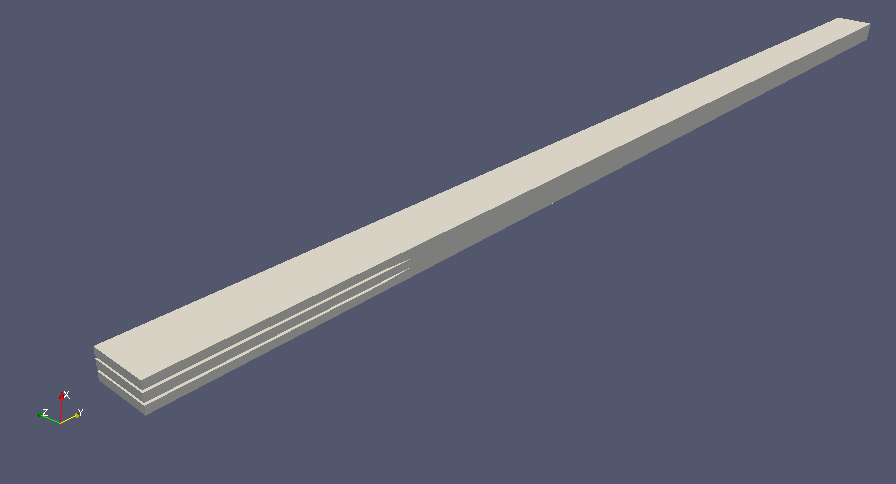Model geometry (entire)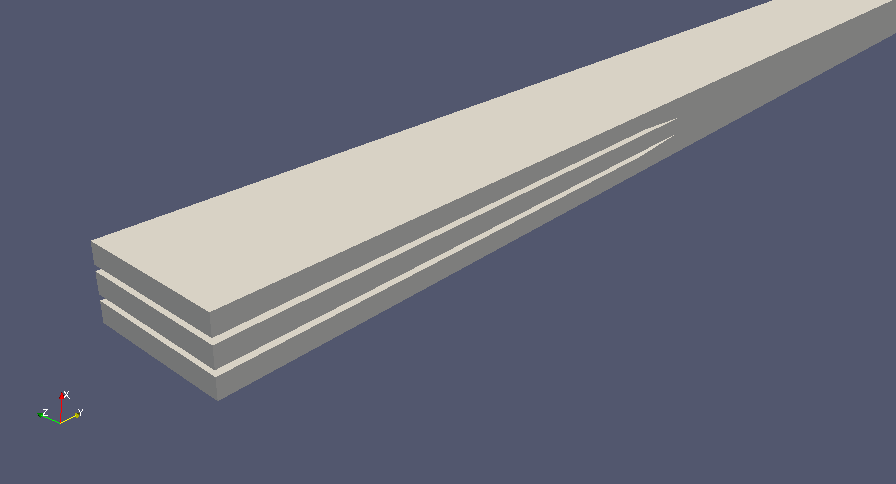Model geometry (around inlets)

Local time stepping (LTS) is used as a calculation method. The local time stepping is a fast computation method that changes the time step for each mesh according to the mesh size. The local time stepping function is enabled by setting "localEuler" as ddtSchemes in the file "fvSchemes" in the directory "system", as shown below.

```ddtSchemes
{
default         localEuler;
}
```

In the file "fvSolution" in the same directory, parameters such as the maximum width of the time step (maxDeltaT) and the max Courant number (maxCo) are set as follows.

```PIMPLE
{
nOuterCorrectors 1;
nCorrectors     2;
nNonOrthogonalCorrectors 0;
momentumPredictor yes;
rhoMin          0.1;
rhoMax          1.5;

maxCo           2.5;
rDeltaTSmoothingCoeff 0.5;
alphaTemp       1;
maxDeltaT       1;
}
```

In the file "reactingCloud1Properties" in the directory "constant", We set the "phaseChangeModel liquidEvaporation" so that the water will evaporate.

In the file "controlDict" in the directory "system", the following settings are made to output the weighted average of the water volume and temperature at the region "outlet".

```functions
{
surfaceRegion1
{
type            surfaceRegion;
libs            ("libfieldFunctionObjects.so");
writeControl    writeTime;
log             yes;
writeFields     no;
regionType      patch;
name            outlet;
operation       weightedAverage;
weightField     phi;
fields
(
H2O
T
);
}
}
```

The meshes are as follows, and the number of mesh is 93400.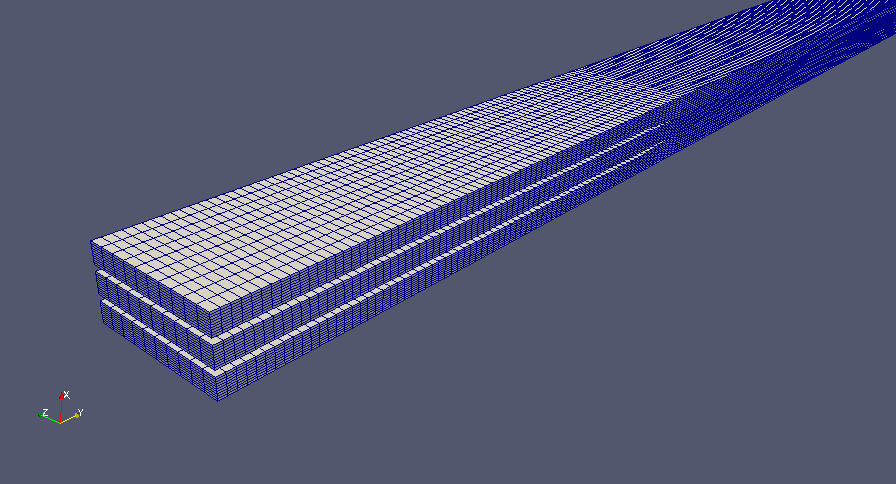Meshes on the inlet side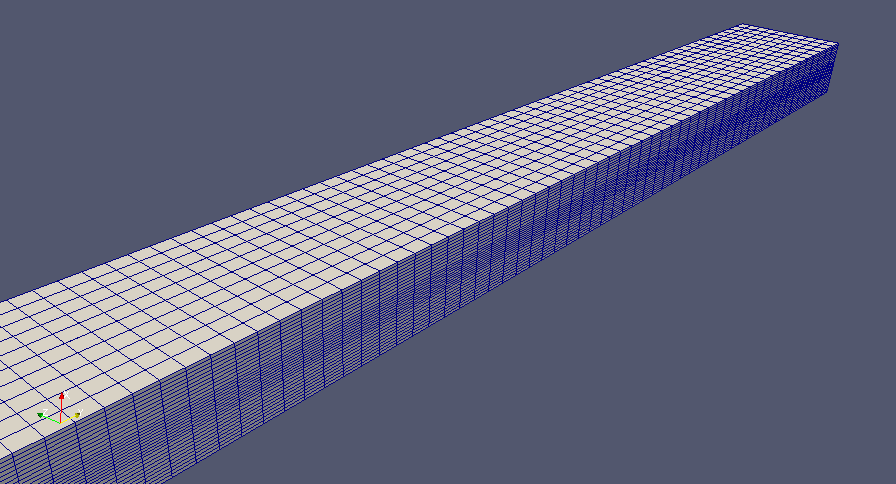Meshes on the outlet side

The calculation results are as follows. All the results are at the final time.

We can see that as it flow through the channel, the water in the center channel where the temperature is higher, evaporates and takes away heat, increasing the (volume) ratio.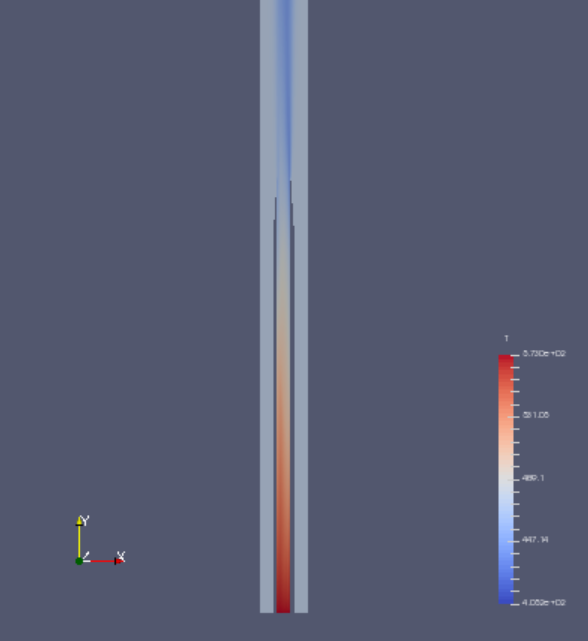Temperature on the inlet side (T)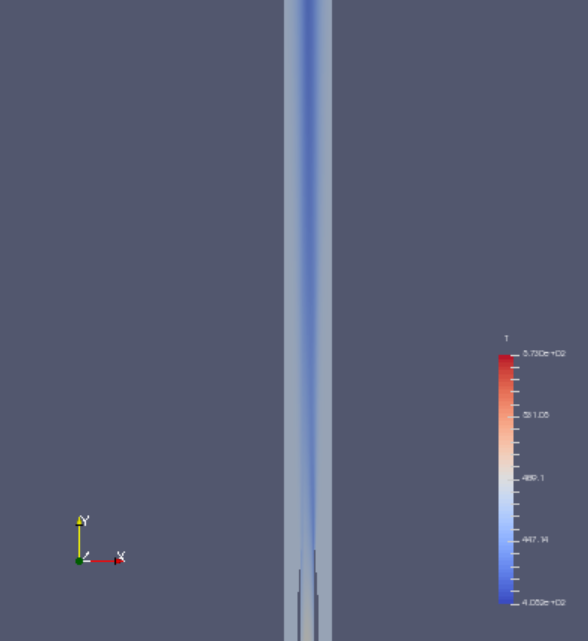Temperature at 3-way junction (T)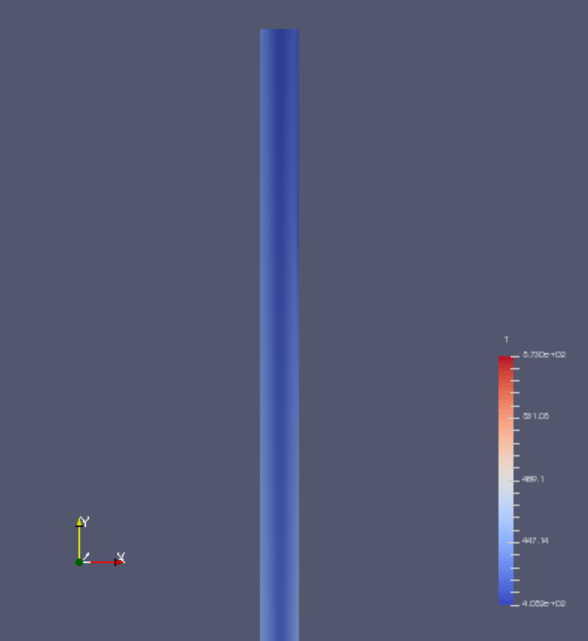Temperature on the outlet side (T)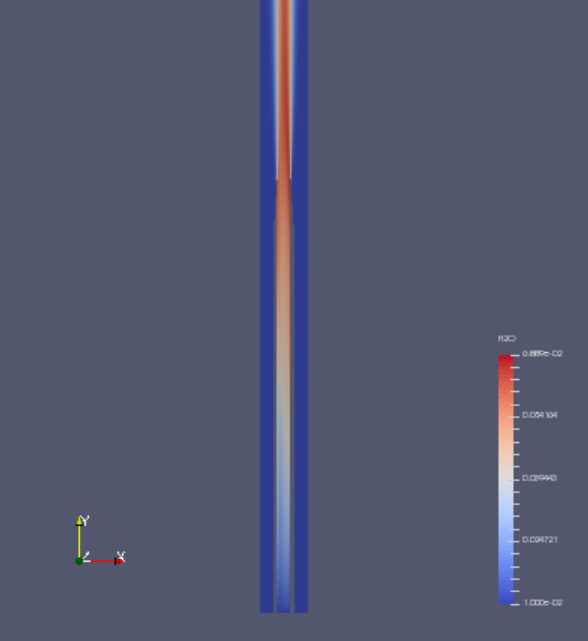Water on the inlet side (H2O)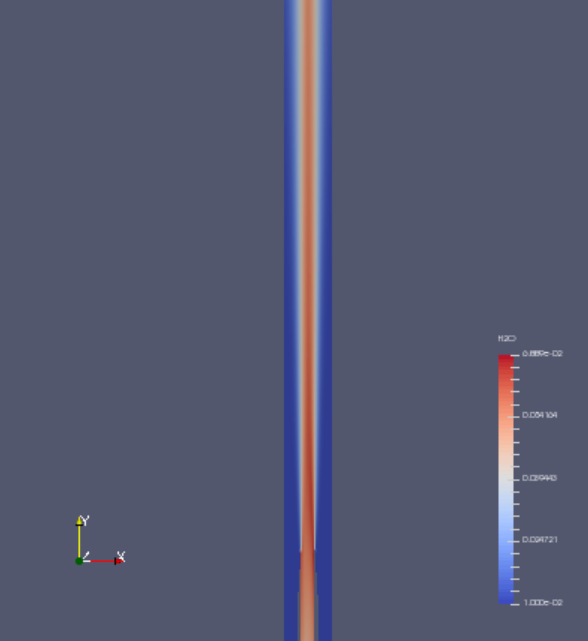Water at 3-way junction (H2O)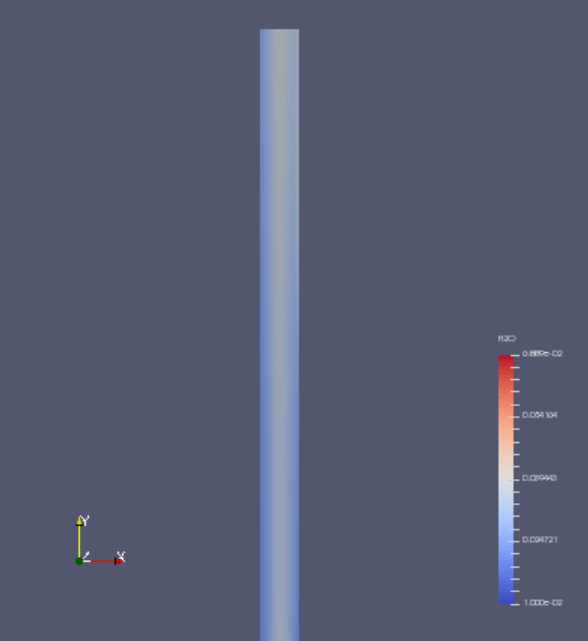Water on the outlet side (H2O)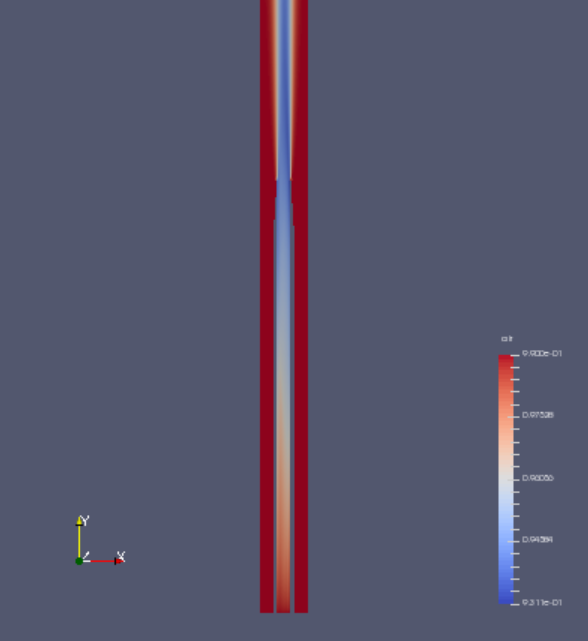Air on the inlet side (air)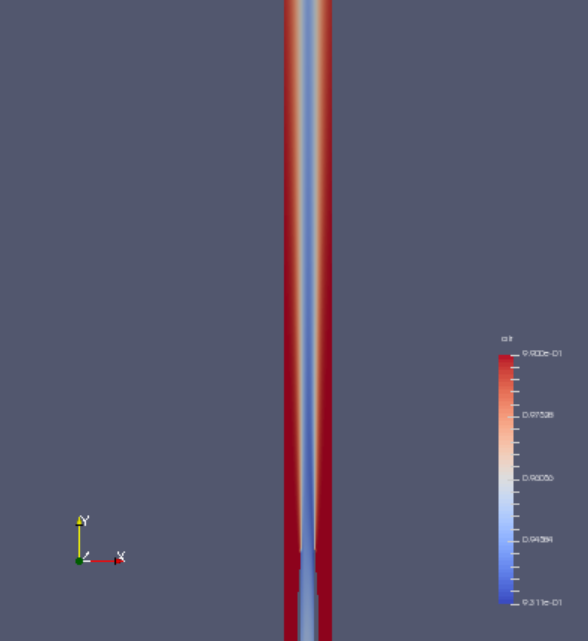Air at 3-way junction (air)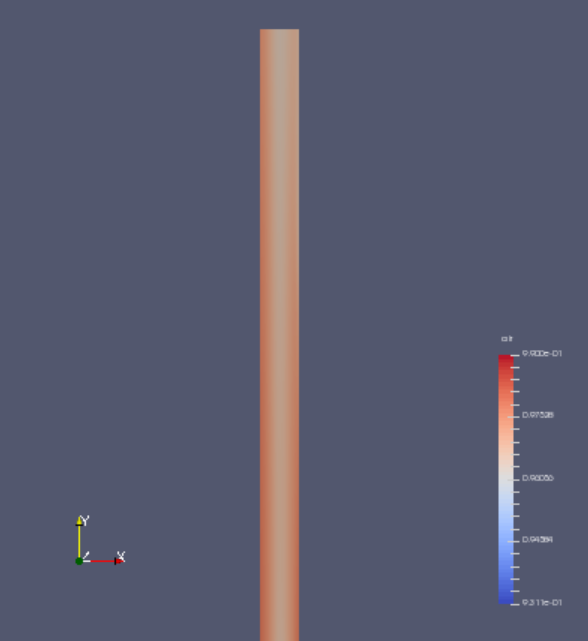Air on the outlet side (air)

The weighted average of water volume and temperature in the outlet region is as follows.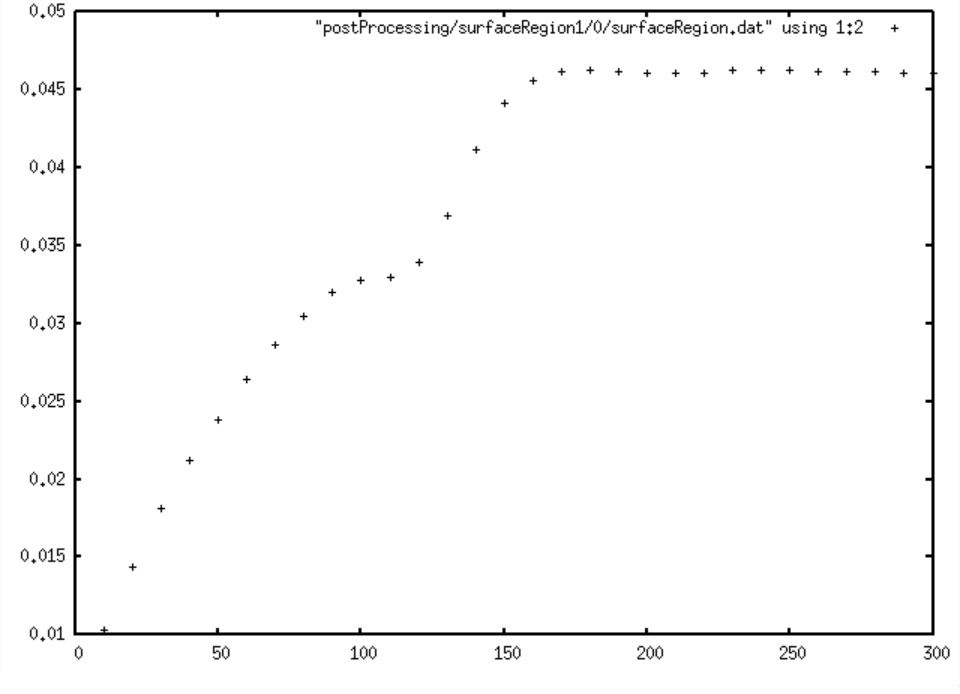Weighted average of water volume at each calculation cycle (H2O)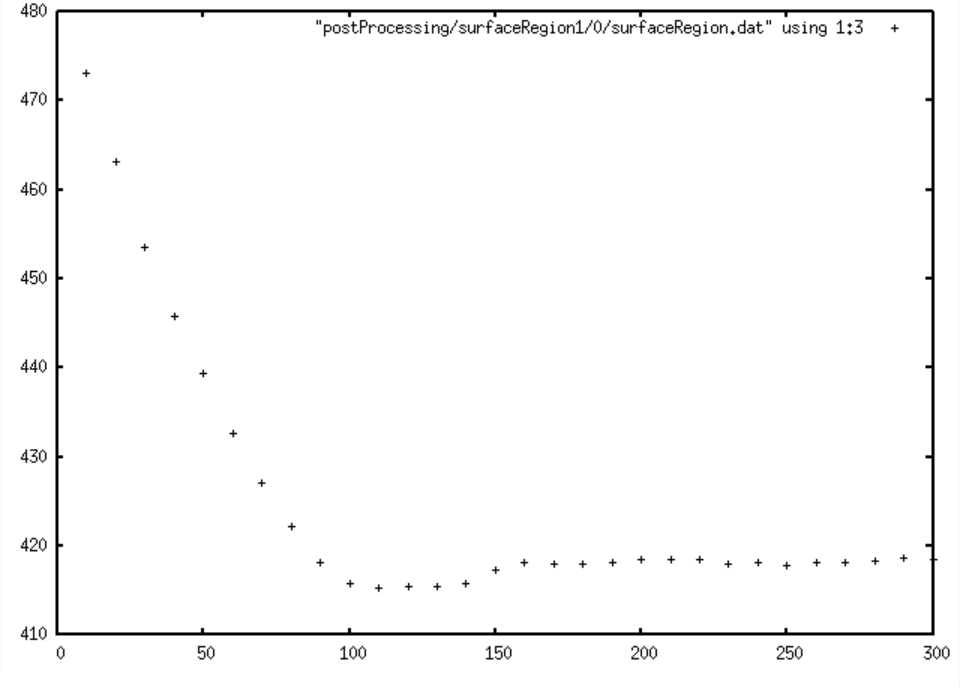Weighted average of temperature at each calculation cycle (T)

## Commands

cp -r \$FOAM_TUTORIALS/lagrangian/reactingParcelFoam/verticalChannelLTS verticalChannelLTS
cd verticalChannelLTS

blockMesh

reactingParcelFoam

paraFoam

gnuplot
gnuplot>plot "postProcessing/surfaceRegion1/0/surfaceRegion.dat" using 1:2 lc rgb "black"
gnuplot>plot "postProcessing/surfaceRegion1/0/surfaceRegion.dat" using 1:3 lc rgb "black"

We use potentialFoam to create the initial conditions in advance.

## Calculation time

5 minutes 59.06 seconds *Single, Inter(R) Core(TM) i7-2600 CPU @ 3.40GHz 3.40GHz